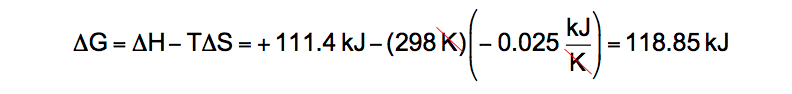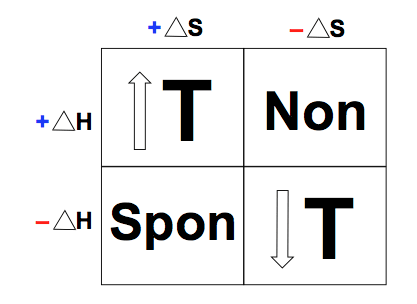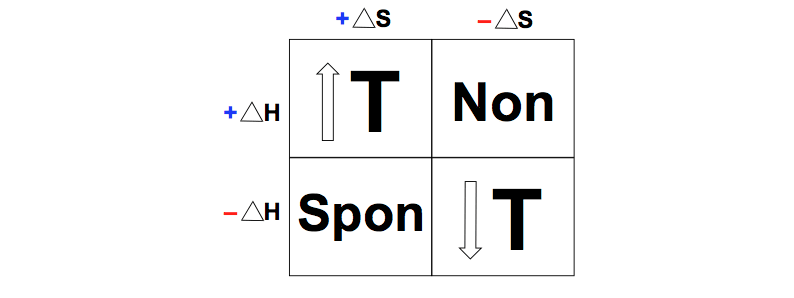Clutch Prep is now a part of Pearson
Ch. 17 - Chemical ThermodynamicsWorksheetSee all chapters

# Gibbs Free Energy

See all sections
Sections
Spontaneous Reaction
First Law of Thermodynamics
Entropy
Gibbs Free Energy
Second and Third Laws of ThermodynamicsJules Bruno

Gibbs Free Energy is used to determine the amount of reusable energy in a thermodynamic system (chemical reaction). Its value can help to determine the direction a reaction takes in order to reach spontaneity.

Gibbs Free Energy, Spontaneity and Entropy

According to the 2nd Law of Thermodynamics, the entropy of the universe always increases for a spontaneous process. This is illustrated by the equation:Second Law of Thermodynamics

Gibbs Free Energy is a thermodynamic property that can be used in determining the direction of a spontaneous process at either constant temperature (isothermal) or constant pressure (isobaric). By rearranging the 2nd Law of Thermodynamics we obtain the following equation to define Gibbs-Free Energy:

ΔGº = ΔHº-TΔSº

PRACTICE: For a particular reaction, ΔH = + 111.4 kJ and ΔS = – 25.0 J/K. Calculate ΔG for this reaction at 298 K. What can be said about the spontaneity of the reaction at 298 K?

a) ΔG = – 103.95 kJ; The system is at equilibrium

b) ΔG = + 118.85 kJ; The system is spontaneous in the reverse direction.

c) ΔG = + 118.85 kJ; The system is spontaneous as written.

STEP 1: Change the units of entropy from joules (J) to kilojoules (kJ) because both enthalpy (ΔH) and Gibbs-Free energy (ΔG) are in kJ.Entropy Value Conversion

STEP 2: Plug in your values into the Gibbs-Free Energy formula to obtain your answer.Calculating Gibbs Free Energy

STEP 3: Whenever Gibbs-Free energy (ΔG) is a positive value this means that the chemical reaction will be non-spontaneous in the forward direction. However chemical reactions always wish to move in the direction that makes them spontaneous. This means the reaction will move in the reverse direction to become spontaneous. Option B is the correct answer.

Spontaneity & Temperature

The researchers Josiah Willard Gibbs and Hermann von Helmholtz theorized that at constant pressure there is a strong correlation between temperature and Gibbs-Free Energy. If we know the signs of enthalpy (ΔH) and entropy (ΔS) then varying temperatures can predict spontaneity.Gibbs Free Energy Table & Spontaneity

+ΔH  +ΔS

When both enthalpy and entropy are positive values then the reaction becomes more spontaneous (ΔG < 0) as the temperature increases.

+ΔH ΔS

When enthalpy is positive and entropy is negative then the reaction will always be non-spontaneous (ΔG > 0) at all temperatures.

ΔH +ΔS

When enthalpy is negative and entropy is positive then the reaction will always be spontaneous (ΔG < 0) at all temperatures.

ΔH ΔS

When both enthalpy and entropy are negative values then the reaction becomes more spontaneous (ΔG < 0) as the temperature decreases.

PRACTICE: Which statement best describes the following endothermic reaction?

C2H6(g) → C2H4(g) + H2(g)

This reaction is ________.

a) spontaneous at all temperatures

b) spontaneous only at a high temperature

c) spontaneous only at a low temperature

d) nonspontaneous at all temperatures

STEP 1: Identify the sign of enthalpy (ΔH).

We are told that the chemical reaction is exothermic. This means that enthalpy is a negative value.

STEP 2: Identify the sign of entropy (ΔS).

Entropy represents chaos or disorder. As a result of one reactant molecule producing multiple product molecules there is an increase in entropy. This means that entropy is a positive value.

STEP 3: Match the signs of enthalpy (ΔH) and entropy (ΔS) to our spontaneity grid.Temperature and Gibbs Free Energy

Since enthalpy is negative and entropy is positive the reaction will be spontaneous (ΔG < 0) at all temperatures. Option A is the correct answer.

Endergonic and Exergonic Reactions

Exergonic reactions have a release of energy that results in the formation of less-energetic products with greater stability. In addition the Gibbs Free Energy value will be negative, the process will be spontaneous and the forward direction will be favored in the formation of products.Exergonic Energy Diagram

Endergonic reactions deal with the absorption of energy that results in the formation of more-energetic and less stable products. In addition the Gibbs Free Energy value will be positive, the process will be non-spontaneous, and the reverse direction will be favored in the formation of reactants.Endergonic Energy Diagram

At equilibrium there is no net change in the energy between reactants and products. This results in a Gibbs Free Energy value equal to zero and no direction is predominantly favored.Energetically Neutral Diagram

Gibbs Free energy is at the center of our understanding of spontaneity, but isn’t the only useful variable. Other variables such as the equilibrium constant (K)cell potential (ΔE), entropy (ΔS), and the internal energy (ΔU) of a process can also be examined.Jules Bruno

Jules felt a void in his life after his English degree from Duke, so he started tutoring in 2007 and got a B.S. in Chemistry from FIU. He’s exceptionally skilled at making concepts dead simple and helping students in covalent bonds of knowledge.